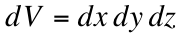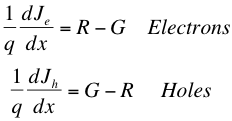# V. Equations of Device Physics

Now that we have described many of the properties of semiconductors, we can give a set of equations that govern that operation of semiconductor devices and which will provide the ideal characteristics for solar cells. We will examine the following sets of equations in one dimension; the three-dimensional form for these equations is similar except that the derivatives are replaced by divergence operators for vector quantities (such as electric field) and by the gradient operator for scalar quantities (such as potentials or concentration gradients)1.

## Current Density Equations (Review)

We have already studied the current-density equations as they appear below in the diffusion section, but it is good to review what we know in consolidated form. We have that the total current density of electrons and holes in doped semiconductors includes both the drift and diffusion currents. The drift of the charge carrier depends on the mobility of charge carriers in the material, the strength of the electric field, and the concentration of charge carriers. The diffusion current, on the other hand, depends on the diffusion constant (Einstein’s relation, which in turn depends on mobility) and the concentration gradient (the concentration difference relative to position). The equations for the current density of electrons and holes are given below.## Poisson's Equation

This next relation comes from electrostatics, and follows from Maxwell’s equations of electromagnetism. Poisson’s equation relates the charge contained within the crystal with the electric field generated by this excess charge, as well as with the electric potential created. The equation is given below1:where the left term is the negative second derivative of the potential ϕ, E is the strength of the electric field, ρ is the charge density in the crystal, and ε is the material’s permittivity (an intrinsic property measuring the resistance to the forming of an electric field). So, this means that a higher change in the electric field relative to position (in a given material) signifies a higher charge density. Note: this equation can also be written as the charge density divided by the relative permittivities, so that we have ε=εrε0 where εr is the relative permittivty of the material and ε0 is the permittivity of a vacuum.

It is important then to look at charge density and determine what this is in terms of the previously discussed concentrations of charge carriers. We know that because electrons in the conduction band contribute negative charge and holes in the valence band contribute a positive charge, donor atoms that have been ionized (lost an electron) have an excess positive charge and ionized acceptor atoms have negative charge. This then gives us the most basic form for charge density in a doped semiconductor2:where q is the fundamental unit of charge, p and n are the concentrations of holes and electrons respectively, and ND+ and NA- are the concentrations of ionized donors and acceptors.

It is worth noting that under normal conditions, most donors and acceptors in a doped semiconductor are ionized2, meaning that the last two terms of the above equation are approximately equal to the total concentration of donors, ND, and acceptors, NA, respectively, giving## Continuity Equations

This next set of equations is beneficial for keeping track of particles moving in and out of a given volume of the semiconductor. They are called the continuity equations because they essentially ensure that the particles we identify as passing through a given volume are being generated and recombined appropriately in order to account for changes in flow rate within the volume. So, given an infinitesimally small volumewhere the product on the right is of three infinitesimally small units in three dimensions, we can say that (restricting ourselves to one dimension for particle flow) the difference in particle flows in this unit volume must realistically be equal to the difference between the generation and recombination of these particles. Otherwise, we would have particles appearing seemingly from nowhere! This then gives us the following condition1:where F is the function in terms of position x representing particle flow, G is the “generation rate”, and R is the “recombination rate”.

Now, if we convert these particle flows into electrons and holes and use our knowledge of the current density, we can relate the current density with the generation and recombination of charge carriers. Taking sign into account, we obtain1where Je and Jh are the current densities for electrons and holes respectively and q is the elementary unit of charge.Above we have an illustration of the changes in concentration of charge carriers (and thus changes in current density) that can occur within a given volume; this must be taken into account when considering the flow of carriers through this region.

## References

1. Goetzberger, Adolf et.al. Crystalline Silicon Solar Cells. Chichester: John Wiley & Sons Ltd., 1998.

2. Green, Martin A. Solar Cells: Operating Principles, Technology, and System Applications. Englewood Cliffs: Prentice-Hall, Inc., 1982. Full book ordering information at www.pv.unsw.edu.au.